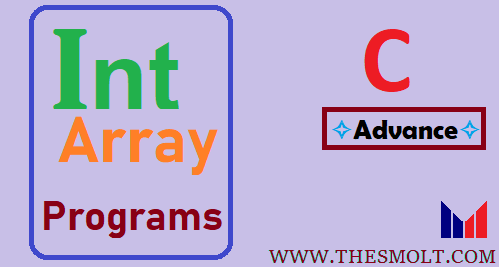# How do you write int Array in C programming examples

## Array in C programming examples

Hello, student today we learn about the intArray Program in C, Previous we already learned 2D (Two Dimensional Array) and Many different Array Related Programs.

## How do you write an int array

```#include <stdio.h>

#include <conio.h>
int main()
{

printf("Welcome to Thesmolt\n\n");

int size_of_array, iteration;
int *array;//defining array as pointer
printf("Enter the size of the array: ");
scanf("%d", &size_of_array);
printf("Enter the elements of the array:\n");
for(iteration = 0 ; iteration < size_of_array ; iteration ++ )
{
scanf("%d", &array[iteration]);
}
printf("The array is:\n");
for(iteration = 0 ; iteration < size_of_array ; iteration ++ )
{
printf("The element at index %d is: %d\n",iteration, array[iteration]);
}
return 0;
}```
Output

Welcome to Thesmolt

## Example of Int array## Array declaration in C

int *array; this is not array unless you dynamically assign memory to it, int *array means it can point to a memory address where int is stored. .    For Example:-

(a; //int  Array

END